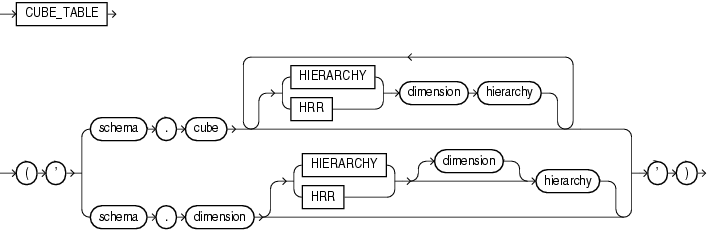CUBE_TABLE

SyntaxDescription of the illustration cube_table.eps

Purpose

CUBE_TABLE extracts data from a cube or dimension and returns it in the two-dimensional format of a relational table, which can be used by SQL-based applications.

The function takes a single VARCHAR2 argument. The optional hierarchy clause enables you to specify a dimension hierarchy. A cube can have multiple hierarchy clauses, one for each dimension.

You can generate these different types of tables:

• A cube table contains a key column for each dimension and a column for each measure and calculated measure in the cube. To create a cube table, you can specify the cube with or without a cube hierarchy clause. For a dimension with multiple hierarchies, this clause limits the return values to the dimension members and levels in the specified hierarchy. Without a hierarchy clause, all dimension members and all levels are included.

• A dimension table contains a key column, and a column for each level and each attribute. It also contains a MEMBER_TYPE column, which identifies each member with one of the following codes:

• L - Loaded from a table, view, or synonym

• A - Loaded member and the single root of all hierarchies in the dimension, that is, the "all" aggregate member

• C - Calculated member

All dimension members and all levels are included in the table. To create a dimension table, specify the dimension without a dimension hierarchy clause.

• A hierarchy table contains all the columns of a dimension table plus a column for the parent member and a column for each source level. It also contains a MEMBER_TYPE column, as described for dimension tables. Any dimension members and levels that are not part of the named hierarchy are excluded from the table. To create a hierarchy table, specify the dimension with a dimension hierarchy clause.

CUBE_TABLE is a table function and is always used in the context of a SELECT statement with this syntax:

SELECT ... FROM TABLE(CUBE_TABLE('arg'));

Examples

The following examples require Oracle Database with the OLAP option and the GLOBAL sample schema. Refer to Oracle OLAP User’s Guide for information on downloading and installing the GLOBAL sample schema.

The following SELECT statement generates a dimension table of CHANNEL in the GLOBAL schema.

SELECT dim_key, level_name, long_description, channel_total_id tot_id,
channel_channel_id chan_id, channel_long_description chan_desc,
total_long_description tot_desc
FROM TABLE(CUBE_TABLE('global.channel'));

DIM_KEY      LEVEL_NAME LONG_DESCRIPTION TOT_ID CHAN_ID CHAN_DESC    TOT_DESC
-----------  ---------- ---------------- ------ ------- ------------ -------------
CHANNEL_CAT  CHANNEL    Catalog          TOTAL  CAT     Catalog      Total Channel
CHANNEL_DIR  CHANNEL    Direct Sales     TOTAL  DIR     Direct Sales Total Channel
CHANNEL_INT  CHANNEL    Internet         TOTAL  INT     Internet     Total Channel
TOTAL_TOTAL  TOTAL      Total Channel    TOTAL                       Total Channel

The next statement generates a cube table of UNITS_CUBE. It restricts the table to the MARKET and CALENDAR hierarchies.

SELECT sales, units, cost, time, customer, product, channel
FROM TABLE(CUBE_TABLE('global.units_cube HIERARCHY customer market HIERARCHY time calendar'))
WHERE rownum < 20;

SALES      UNITS       COST TIME                       CUSTOMER       PRODUCT     CHANNEL
---------- ---------- ---------- -------------------------- -------------- ----------- -----------
24538587.9      61109 22840853.7 CALENDAR_QUARTER_CY1998.Q1 TOTAL_TOTAL    TOTAL_TOTAL TOTAL_TOTAL
24993273.3      61320   23147171 CALENDAR_QUARTER_CY1998.Q2 TOTAL_TOTAL    TOTAL_TOTAL TOTAL_TOTAL
25080541.4      65265 23242535.4 CALENDAR_QUARTER_CY1998.Q3 TOTAL_TOTAL    TOTAL_TOTAL TOTAL_TOTAL
26258474      66122 24391020.6 CALENDAR_QUARTER_CY1998.Q4 TOTAL_TOTAL    TOTAL_TOTAL TOTAL_TOTAL
32785170      77589 30607218.1 CALENDAR_QUARTER_CY1999.Q1 TOTAL_TOTAL    TOTAL_TOTAL TOTAL_TOTAL
. . .